# C++ Program to Print Number Pattern

In this tutorial you will learn about the C++ Program to Print Number Pattern and its application with practical example.

## C++ Program to Print Number Pattern

In this tutorial, we will learn to create a C++ program that will Print Number patterns using C++ programming.

## Prerequisites

Before starting with this tutorial we assume that you are best aware of the following C++ programming topics:

• Operators in C++ Programming.
• Basic Input and Output function in C++ Programming.
• Basic C++ programming.
• Basic arithmetic operations.
• For loop in C++ Programming.

The C++ language is a very powerful programming language. In C++ programming we can perform many operations with the help of codings. The C++ language is very easy to create any pattern. In this program, we will learn to Print Number Patterns with the help below code.

## Program:-

To Print Number Pattern.

## Output:-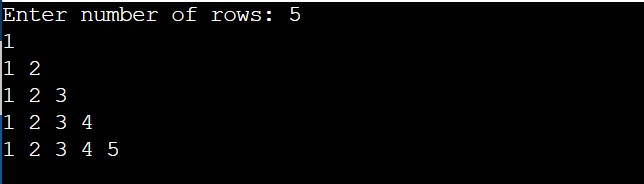In the above program, we have first initialized the required variable.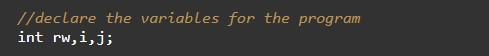• i = it will hold the integer value to control parent for loop.
• j = it will hold the integer value to control child for loop.
• rw = it will hold the integer value.

Taking the input rows from the users.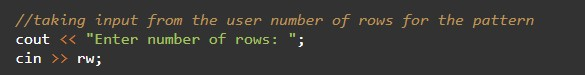Code for the pattern of the number.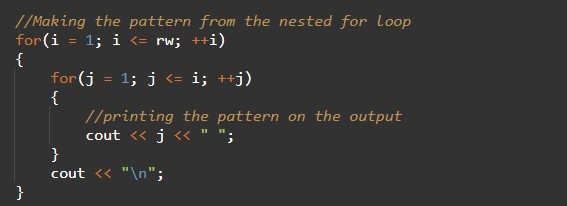Printing the rows of patterns.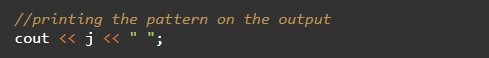In this tutorial we have learn about the C++ Program to Print Number Pattern and its application with practical example. I hope you will like this tutorial.